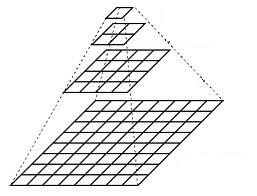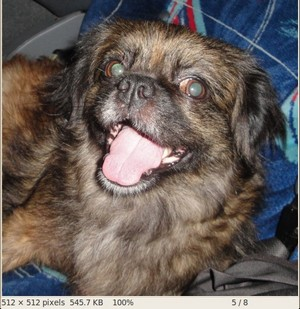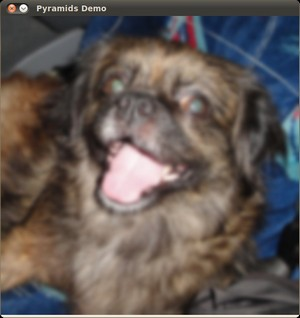OpenCV  4.0.0 Open Source Computer Vision
Image Pyramids

Next Tutorial: Basic Thresholding Operations

## Goal

In this tutorial you will learn how to:

• Use the OpenCV functions pyrUp() and pyrDown() to downsample or upsample a given image.

## Theory

Note
The explanation below belongs to the book Learning OpenCV by Bradski and Kaehler.
• Usually we need to convert an image to a size different than its original. For this, there are two possible options:
1. Upsize the image (zoom in) or
2. Downsize it (zoom out).
• Although there is a geometric transformation function in OpenCV that -literally- resize an image (resize , which we will show in a future tutorial), in this section we analyze first the use of Image Pyramids, which are widely applied in a huge range of vision applications.

### Image Pyramid

• An image pyramid is a collection of images - all arising from a single original image - that are successively downsampled until some desired stopping point is reached.
• There are two common kinds of image pyramids:
• Gaussian pyramid: Used to downsample images
• Laplacian pyramid: Used to reconstruct an upsampled image from an image lower in the pyramid (with less resolution)
• In this tutorial we'll use the Gaussian pyramid.

#### Gaussian Pyramid

• Imagine the pyramid as a set of layers in which the higher the layer, the smaller the size.• Every layer is numbered from bottom to top, so layer $$(i+1)$$ (denoted as $$G_{i+1}$$ is smaller than layer $$i$$ ( $$G_{i}$$).
• To produce layer $$(i+1)$$ in the Gaussian pyramid, we do the following:

• Convolve $$G_{i}$$ with a Gaussian kernel:

$\frac{1}{16} \begin{bmatrix} 1 & 4 & 6 & 4 & 1 \\ 4 & 16 & 24 & 16 & 4 \\ 6 & 24 & 36 & 24 & 6 \\ 4 & 16 & 24 & 16 & 4 \\ 1 & 4 & 6 & 4 & 1 \end{bmatrix}$

• Remove every even-numbered row and column.
• You can easily notice that the resulting image will be exactly one-quarter the area of its predecessor. Iterating this process on the input image $$G_{0}$$ (original image) produces the entire pyramid.
• The procedure above was useful to downsample an image. What if we want to make it bigger?: columns filled with zeros ( $$0$$)
• First, upsize the image to twice the original in each dimension, with the new even rows and
• Perform a convolution with the same kernel shown above (multiplied by 4) to approximate the values of the "missing pixels"
• These two procedures (downsampling and upsampling as explained above) are implemented by the OpenCV functions pyrUp() and pyrDown() , as we will see in an example with the code below:
Note
When we reduce the size of an image, we are actually losing information of the image.

## Code

This tutorial code's is shown lines below.

## Explanation

Let's check the general structure of the program:

#### Loop

Perform an infinite loop waiting for user input. Our program exits if the user presses ESC. Besides, it has two options:

• Perform upsampling - Zoom 'i'n (after pressing 'i')

We use the function pyrUp() with three arguments:

• src: The current and destination image (to be shown on screen, supposedly the double of the input image)
• Size( tmp.cols*2, tmp.rows*2 ) : The destination size. Since we are upsampling, pyrUp() expects a size double than the input image (in this case src).
• Perform downsampling - Zoom 'o'ut (after pressing 'o')

We use the function pyrDown() with three arguments (similarly to pyrUp()):

• src: The current and destination image (to be shown on screen, supposedly half the input image)
• Size( tmp.cols/2, tmp.rows/2 ) : The destination size. Since we are upsampling, pyrDown() expects half the size the input image (in this case src).

Notice that it is important that the input image can be divided by a factor of two (in both dimensions). Otherwise, an error will be shown.

## Results

• The program calls by default an image chicky_512.png that comes in the samples/data folder. Notice that this image is $$512 \times 512$$, hence a downsample won't generate any error ( $$512 = 2^{9}$$). The original image is shown below:• First we apply two successive pyrDown() operations by pressing 'd'. Our output is:• Note that we should have lost some resolution due to the fact that we are diminishing the size of the image. This is evident after we apply pyrUp() twice (by pressing 'u'). Our output is now: